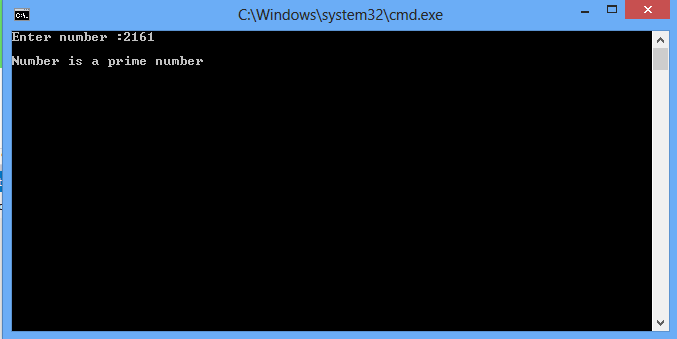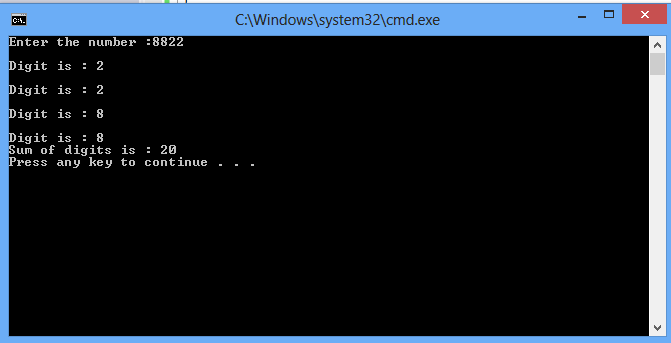# While LOOP

In this part you will learn: 1. While LOOP 2. C syntax 3. Showing output In this tutorial I will teach you how to use while loops in C. We have already covered for loops and now we know what are loops What is a WHILE LOOP ? First of all we will talk about what is while loop. For a loop we need three statement initialization, condition and increment. In a while loop we only write the condition within the round brackets and increment within the body of the loop. We initialize the variable outside the loop. Checking for a prime number Basic Step: Open Dev C++ then File > new > source file and start writing the code below.
`#include<stdio.h>#include<conio.h>`
These are the header files that we need for coding. The main header is stdio.h which provides us with most of the functions for coding in C and the second header files is just for using a function which can pause the screen until the user hits any key.
`int main(){int num,i=2;`
For finding whether a number is prime or not we will simply take input in a number and make a loop counter to test whether it is divisible by numbers from 2 to 1 less than the number if yes then it is a prime number.
`	printf("Enter number :");	scanf("%d",&num);`
Now taking input from the user.
`while ( i<num){	if (num %i==0 ){		printf("\nNumber is not a prime number\n ");		break;	}	i+=1;}`
Now we write the while loop. Note that we already initialized the i variable with 2 ( because we will divide the number from 2 to 1 less than the number in order to find whether the number is prime or not.). After that we write the conditional statement If the remainder of number divided by loop counter is 0 then it is not a prime number. After the condition we increment the variable.
`	if (i== num)		printf("\nNumber is a prime number\n ");	else		printf("\nNumber is not a prime number\n ");	_getch();	return 0;}`
If the loop is finished without the condition becoming true and the incremented value becomes equal to the number then that number is a prime number. Otherwise not. Execute > compile then Execute > run OutputSUM OF DIGITS File > new source file
`#include<stdio.h> int main(){	int num,temp=0,sum=0; 	printf("Enter the number :");	scanf("%d",&num);		}`
For calculating the sum of digits of number we need three variables number, temporary variable and sum. We take the input in number.
`while(num>0)	{		temp = num%10;		sum +=temp; 		printf("\nDigit is : %d\n",temp);              num=num/10;	}	printf("Sum of digits is : %d\n",sum);	return 0;}`
Now we write the loop condition that run until the number is greater than 0 because we will start separating the digits form Least significant to most significant. We take the remainder of number with 10 to separate the digit and simple store it in our temp variable and add that variable to sum until the number is greater than 0. In the end we simply print the sum and return. Execute > compile then Execute > run Output: